# Crop Yield Prediction Using Artificial Neural Network (ANN)

World's 47% population relies on agriculture for a living. With the unpredicted rainfall, increasing temperature, and low-quality fertilizers and pesticides, it is becoming challenging to estimate the production capacity and thus resulting in inefficient use of resources. This blog will discuss the solution to this problem using an Artificial neural network model. So let's first understand how this model will help the farmers.

Farmers usually rent equipment and hire workers according to the farm capacity and the estimated yield of the crop before the actual harvest season. And for all this, they usually take a loan from the bank by signing the contract mentioning the estimated production. A model that predicts accurate yield will be a life saviour for these farmers.

The main focus of this blog will be:

1. To understand the effect of rainfall, temperature, and pesticides on crop yield.
2. How to train and tune an artificial neural network to predict the crop yield.

So let's first understand the features we will consider to predict the crop yield.

#### Effect of Temperature on Yield

Temperature is the primary factor affecting plant growth, thus directly related to crop production. Most crops give maximum yield in moderate temperatures ranging between 15–30 degrees. But the data shows that temperature keeps fluctuating between years, making it hard to predict the crop yield precisely. The graph below shows countries' average temperature over the years (1901–2016).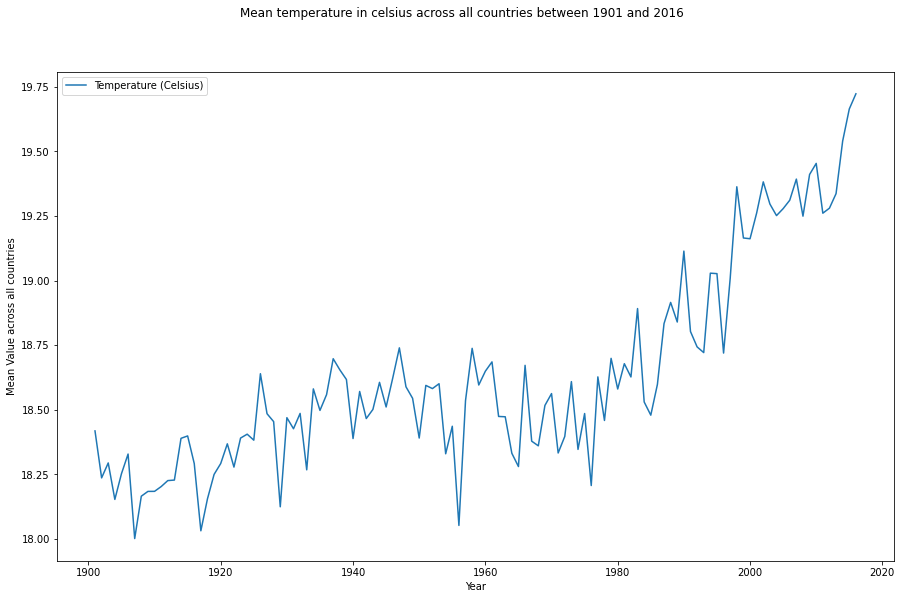#### Effect of Rainfall on Yield

Small and medium farmers depend on rain for irrigation, constituting 78% of the farmer community and producing only 33% of the total yield. Rainfall is one of the most unstable weather parameters, and predicting yield with so much fluctuation in the rain per year is challenging. Here is the graph of rainfall from 1901 to 2016.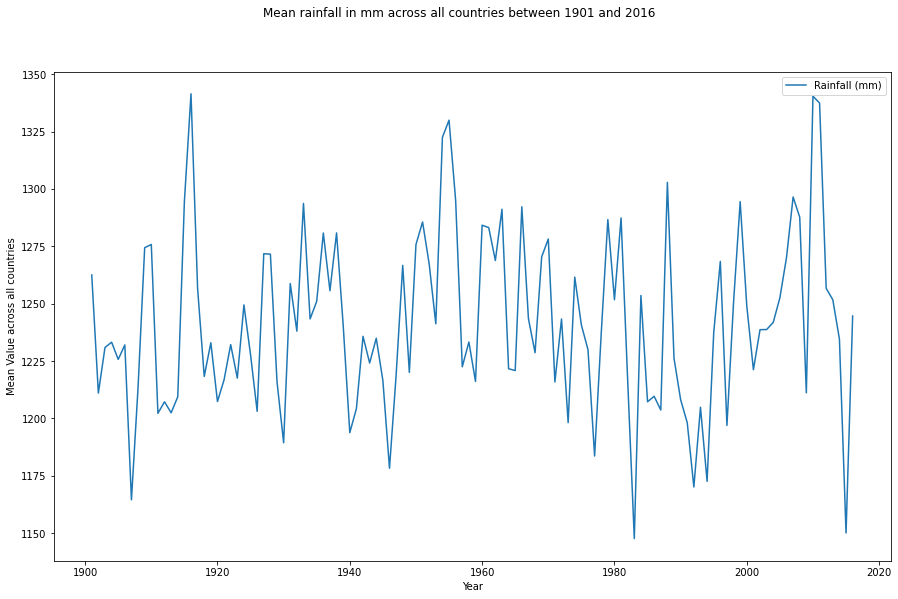#### Effect of Pesticides on Yield

Using fertilizers and pesticides indicates chemical usage in crop production, thus creating a bad image. But with the challenging weather conditions and increasing demand for agriculture, they became mandatory for crops. The usage of pesticides is increasing per year at a great rate, and we can do nothing but consume them through food.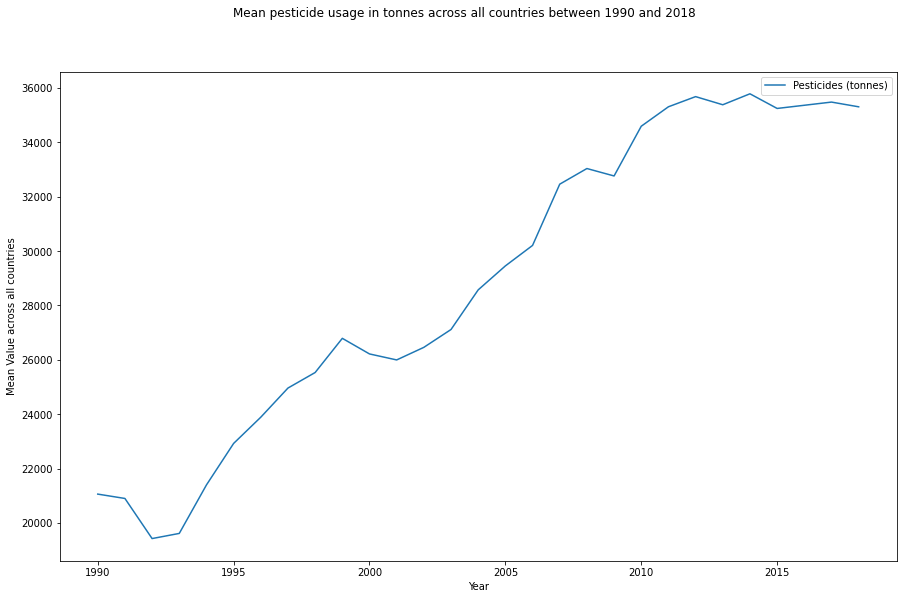Considering all these features, let's build a neural network model to predict the yield of crops.

### Building ANN model

We will follow the standard steps to build this neural network model: download the dataset, clean it, do some data exploration, make it feedable to the model, and finally, build the model.

To begin, we will use Kaggle's Crop Yield Prediction dataset, having five CSV files: pesticides.csv, rainfall.csv, temp.csv, yield.csv, and yield_df.csv. The last CSV file is the combined data of the other four files, so that we will use this dataset for the model.

``````import numpy as np
import pandas as pd

Following the next step, we will see what pattern the data follows and what we can infer by seeing it for once.

#### Step 2: Data Exploration

Before discussing this step, let's have a look at the data.

``````Unnamed: 0    Area    Item         Year     hg/ha_yield     average_rain_fall_mm_per_year   pesticides_tonnes   avg_temp
O Albania   Maize        1990       36613                   1485.0                     121.0             16.37
1 Albania   Potatoes     1990       66667                   1485.0                     121.0             16.37
2 Albania   Rice, paddy  1990       23333                   1485.0                     121.0             16.37
3 Albania   Sorghum      1990       12500                   1485.0                     121.0             16.37
4 Albania   Sovbeans     1990       7000                    1485.0                     121.0             16.37``````

Some changes can be made in the dataset, like removing the unnamed column and renaming the columns Area, hg/hayield, and averagerainfallmmperyear for easy handling.

``````yield_df.rename({'Area':'Country','hg/ha_yield':'Yield (hg/ha)','average_rain_fall_mm_per_year':'Rainfall (mm)'},axis=1,inplace=True)
yield_df.drop('Unnamed: 0',axis=1,inplace=True)``````

One more thing we can see is the correlation between the features. Because we generally build neural networks when the features are not strongly correlated or do not show a linear connection.

#### Heatmap

A heatmap or correlation matrix shows how features are related to each other and can efficiently study their effect on the target. Values close to 1 show a strong positive correlation, while values near -1 show a strong negative correlation.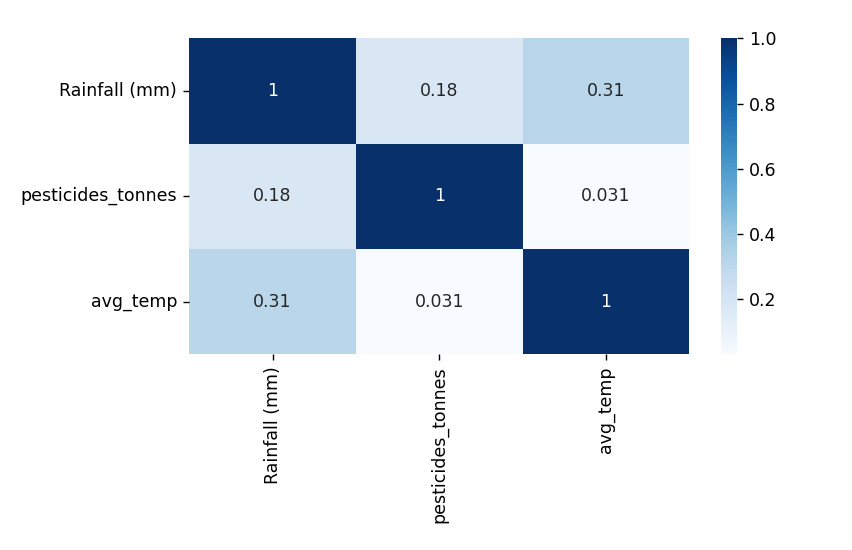The property of a perfect dataset is that features should not correlate with each other to reduce biases. As we can see from the above heatmap, features are not strongly correlated. And if they are, then we drop one of that features.

After knowing in and out of the dataset, it's time to remove any discrepancies if present.

#### Step 3: Data Preprocessing

It is a mandatory step in any data science field, whether data analysis, machine learning, or deep learning. We will perform these steps one by one in this section.

#### Check for Null values

Null values hamper the learning of the model because they might add up to the noise and lead to biased decisions. So we will first check for null values; if they are present, we will remove them.

``````yield_df.isnull().sum()

#####################
'''
Unnamed: 0                     0
Area                           0
Item                           0
Year                           0
hg/ha_yield                    0
average_rain_fall_mm_per_year  0
pesticides_tonnes              0
avg_temp                       0
dtype: int64

'''``````

As we can see, there are no NULL values, so we are ready to move forward.

#### Handling Categorical Columns

Before applying any numerical operation to the data, we need to convert the categorical values of the columns into numeric form. We convert these values into various encoding schemes like one-hot and label encoding.

``````from sklearn.preprocessing import LabelEncoder
le=LabelEncoder()

yield_df['Country_encoded']=le.fit_transform(yield_df['Country'])
yield_df['Item_encoded']=le.fit_transform(yield_df['Item'])

yield_df.drop(['Country','Item'],axis=1,inplace=True)``````

The next step is to check whether the data is centralized and within acceptable boundaries. Let's see how.

#### Removing Outliers

Outliers are those data samples that are present far from the other data samples. They drastically affect the learning of the model and manipulate the predictions towards them. There are two common methods to remove outliers:

1. Inter Quartile Range (IQR)
2. Standard deviation.

We will use the IQR method to detect the presence of outliers. IQR is the difference between the third and first quartile, and data points above Q3 + 1.5 IQR and below Q1–1.5 IQR are treated as outliers.

``````for i in yield_df.columns:
q75, q25 = np.percentile(yield_df[i], [75 ,25])
iqr = q75 - q25
min_val = q25 - (iqr*1.5)
max_val = q75 + (iqr*1.5)

yield_df=yield_df[(yield_df[i]<max_val)]
yield_df=yield_df[(yield_df[i]>min_val)]``````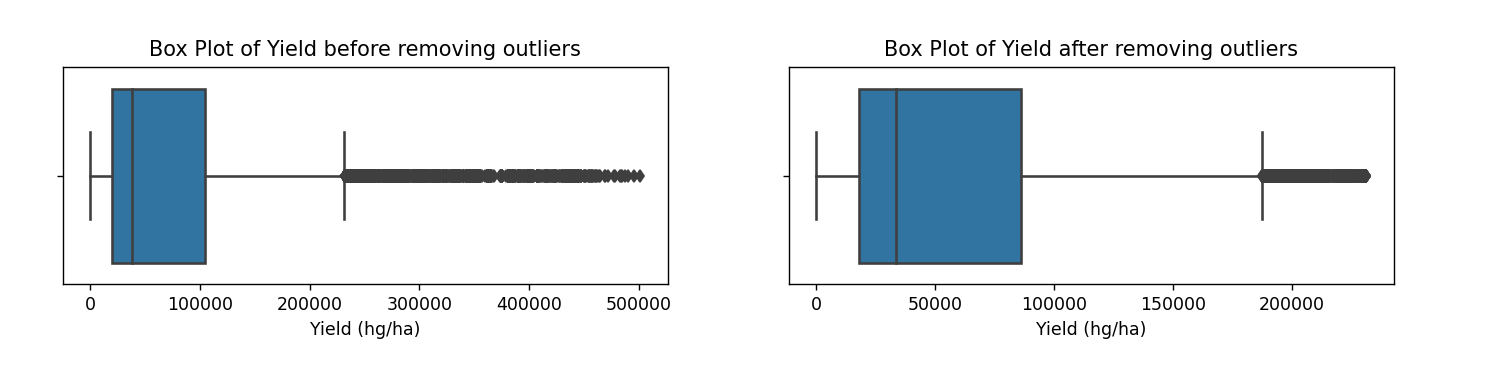The plot shows that there are still some outliers in the dataset and thus proves that standard definitions are not always true. We can set the interquartile range according to the dataset and deal with the remaining outliers. Try changing it. It will be fun!

Now comes the most debatable step in data science, "Feature Scaling". It's been a decade, and we are still unsure about it. Some say feature scaling is good for the model, some say it is unnecessary. But we are here with a clear explanation of why feature scaling is essential.

#### Feature Scaling

These two reasons can explain the need for feature scaling:

1. Scaling features can improve the optimization process by smoothing the gradient descent flow.
2. If the features are not scaled, the algorithm may be biased towards the features with values higher in magnitude.

The mathematical intuition behind these reasons is explained in this blog. Please have a look.

``````Y=yield_df['Yield (hg/ha)']
X=yield_df.drop('Yield (hg/ha)',axis=1)

from sklearn.preprocessing import MinMaxScaler
min_max_scaler=MinMaxScaler()
X_scaled=pd.DataFrame(min_max_scaler.fit_transform(X))``````

Now it's time to learn how to define the neural network model.

### Step 4: Model Formation

For any neural network model, six things are essential to decide.

1. Number of layers
2. Number of neurons in each layer
3. Activation function for each layer
4. Loss function
5. Optimization algorithm
6. Epoch value

What if I say no one can guess these six things ideally in one go? Sounds bizarre. But this is true. To make it easy, I will share my experience building this model and tuning all the hyperparameters to reach a good accuracy score.

#### Deciding the number of layers

First, I started with four layers: one input layer, two hidden layers, and one output layer. The dataset was large, and the temperature, rainfall, and pesticide consumption followed an irregular pattern.

#### Deciding the number of neurons in each layer

We usually specify the number of neurons in the power of two for easy inside-the-layer computations. So I started with the (8, 8, 8, 1) configuration of neurons. There is no thumb rule that one should select the number of neurons in the power of two, but it's good to follow a rule rather than choosing some random value.

#### Deciding the activation function for each layer

We use a linear activation function in the output layer for a regression problem. And it is advised to start with the ReLu activation function for hidden layers and can change them later on to compare the performance of the model. So I used ReLu for the input, two hidden layers, and the linear activation function for the output layer.

#### Selecting the loss function and optimization algorithm

There are several choices for loss function for regression problems like MSE, MAE, RMSE, and Huber loss. I chose MSE as a loss function.

Now, for choosing the optimization algorithm, we should ask whether the dataset is big or small, our system's computational power and whether we want to tune the learning rate manually. After this, we will determine which optimization algorithm is perfect for our model. I used Adam (Adaptive Moment Estimation) algorithm because it is swift and converges quickly.

#### Deciding the Epoch value

Epoch value decides how often the model will be trained over the whole dataset. In machine learning, we usually train the model only once, but in the neural network, the model is trained many times to learn complex patterns. I typically start with an epoch value of 1000 to explore more.

Now let's quickly summarise these steps in the code.

``````x_train,x_test,y_train,y_test=train_test_split(X_scaled,Y,test_size=0.2,random_state=42)

neural_regressor = tf.keras.models.Sequential()

plot_data = neural_regressor.fit(x_train,y_train, epochs=600,)``````

### Tuning the neural network model

If one closely observes the code, the values of the six parameters mentioned differ from what I started with. Let's see how I reached this final configuration of the neural network model.

#### Tuning the number of layers and number of neurons

I started with four layers with neuron configuration (8, 8, 8, 1), and the final structure is three layers with (12, 12, 1) neurons in each layer. I trained the model with different numbers of neurons, keeping the number of layers four and observed that the neurons' (12, 12, 8, 1) configuration works well. Then I removed the layer with eight neurons and trained the model again, and observed that this time model performed better than before. Why add more hidden layers if the model performs well with a less complex configuration?

#### Deciding the activation function

ReLu worked fine with the layers but out of curiosity, I decided to change the activation function to SeLu and to my surprise model converged fast, and the r2 score increased by 4 per cent. R2 score is an evaluation metric for regression models. Let's see why SeLu performed better than ReLu.

#### ReLu v/s SeLu

ReLu stands for Rectified Linear Unit, and to understand the working of this activation function, we must understand what back-propagation is. It is a process in the neural network which enables backward learning and adjusts the weights of nodes. Weights are updated after every epoch by calculating the gradient. Let's see how ReLu and SeLu help in this process.

ReLu follows a simple rule, it vanishes the negative values and gives linear output for positive values.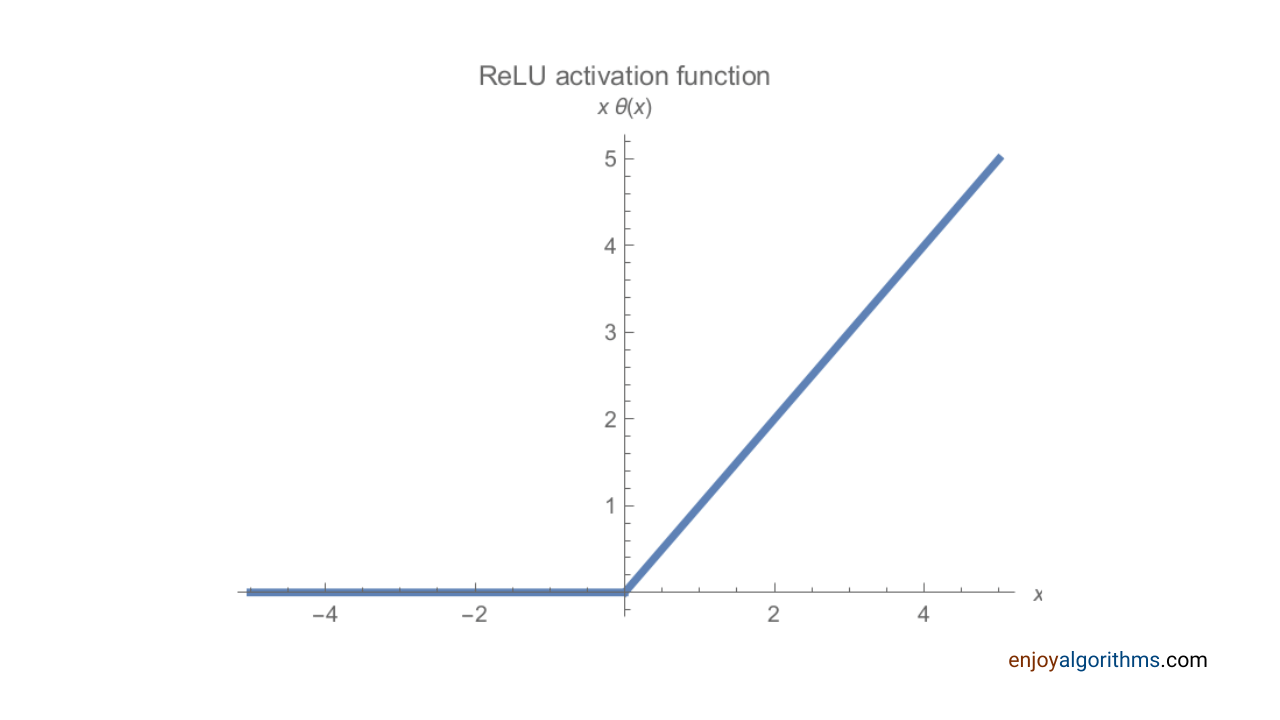Advantage: ReLu accelerates the convergence of the gradient and is computationally efficient because it activates only a few neurons accepting positive inputs.

Disadvantage: It experiences the Dying ReLu problem: getting trapped in a dead state. The weights for some neurons do not get updated, which results in dead neurons.

With time ReLu has been modified to enhance its performance and solve the dying ReLu problem. One of its modified versions and the most efficient is the Scaled Exponential Linear Unit (SeLu).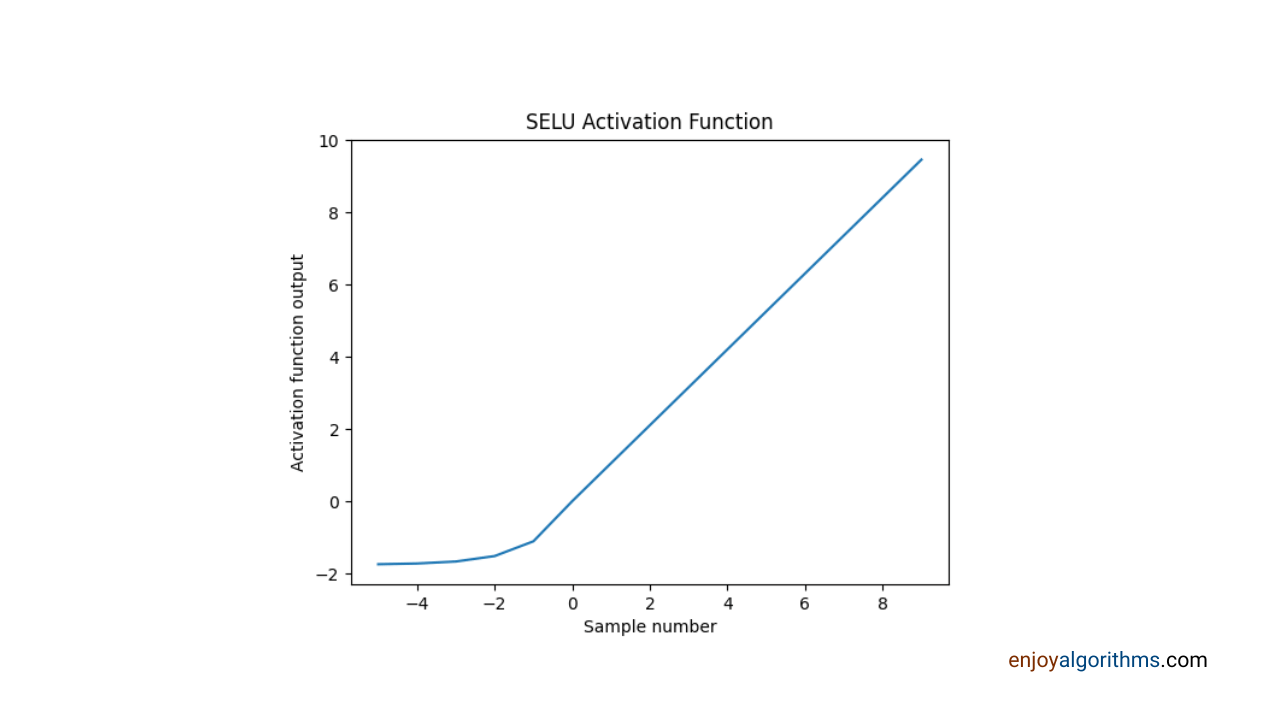1. It is a self-normalizing activation function, meaning layers preserve mean and variance from the previous layer.
2. It is more effective in solving the vanishing gradient problem.

Research on SeLu is still ongoing, and we look forward to it as a solution to the trade-off between solving the dying neurons problem and the vanishing gradient problem.

#### Tuning the epoch value

The best way to tune the epoch value is to plot the loss curve during the model's training and observe the point from which it remains constant or shows less decrement.

``````loss_train = plot_data.history['mean_absolute_error']

epochs = range(1,601)
plt.plot(epochs, loss_train, 'g', label='Training loss')

plt.title('Training loss')
plt.xlabel('Epochs')
plt.ylabel('Loss')
plt.legend()
plt.show()``````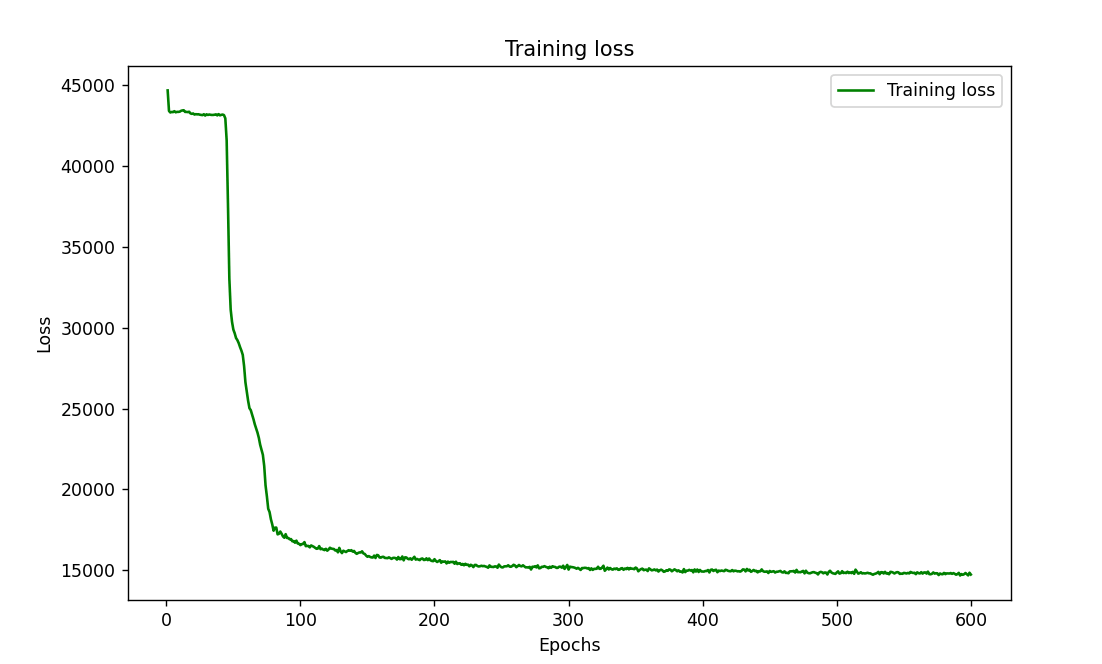I started with an epoch value of 1000, and then after changing the activation function to SeLu, I changed it to 600 because the model converged fast, and from the graph, we can say that after the 150th iteration, there is not much decrement in the loss. I extended the training to 600 iterations to see if it was local or global minima. Now let's check the model performance.

### Step 5: Model Evaluation

"Hard work reaps success, be diligent, and good things will come for you". This is the master formula I follow while building the model. The result will be in our favour if we follow all the data preprocessing steps and select the parameters correctly. To evaluate the performance of this model, we used the R2 score and mean absolute error.

``````y_pred = neural_regressor.predict(x_test)
r2 = r2_score(y_test, y_pred)
print("R2 score: ", r2)
print("MSE: ",mean_squared_error(y_test, y_pred))
print("MAE: ",mean_absolute_error(y_test, y_pred))``````

R2 score: 0.8348491121785119
MSE: 534888584.2619631
MAE: 14721.4678

Instead of all the variations in the features, the model performed well with an R2 score of 83%. I suggest comparing the results before and after tuning the model.

There is one more way to check the fitting of the model through the graph plot. While solving regression problems, we often use this method to get a clear picture of the model fit to the testing and training dataset.

``````ry=range(0,len(x_test))
plt.plot(ry, y_test,color='g')
plt.plot(ry, y_pred,color='k')

plt.show()``````

The below graph is a plot between the actual and predicted values of yield over the testing dataset, and we can say that our model is neither overfitting nor underfitting.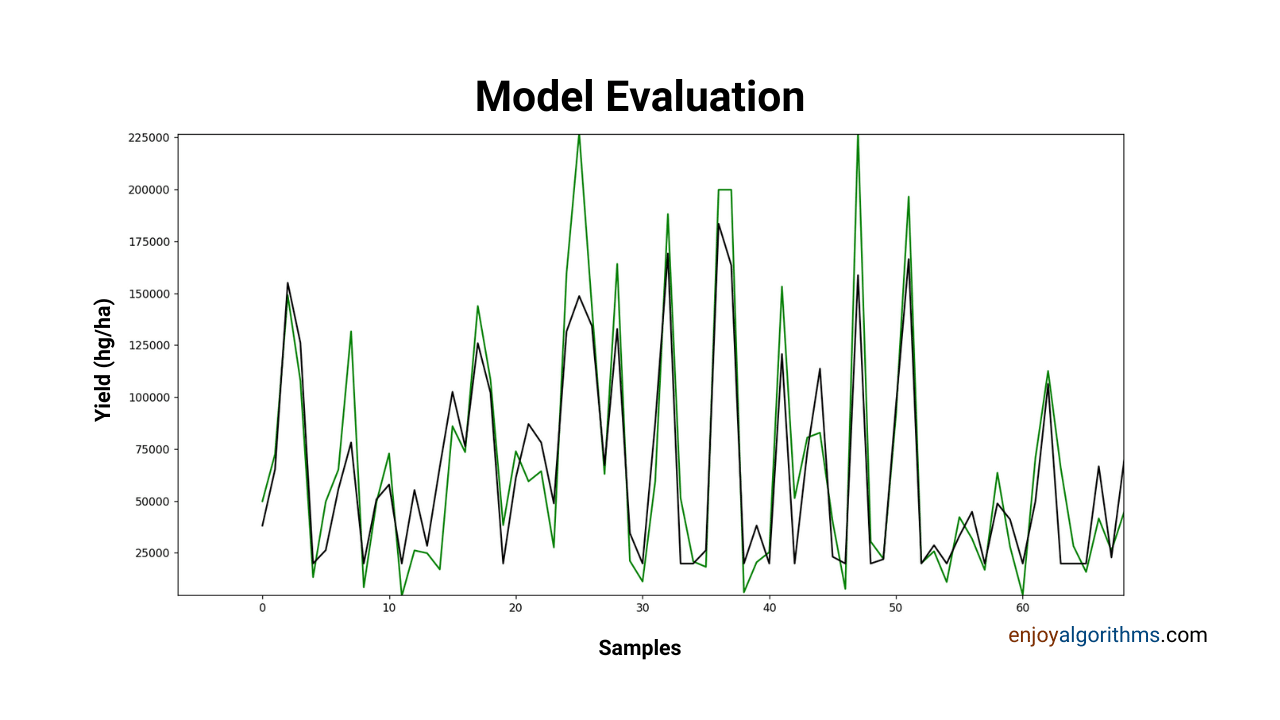That's it for the learning! Explore some more use cases below.

### Company Use Cases

#### Agrograph

This is a startup founded in 2016 with funding of \$500,000 so far and is using machine learning to predict the yield. It uses satellite images with ground-level data provided by farmers, crop lenders, or third-party agencies. Their main concern is to sell this data to crop lenders, crop insurers, and banks so that they can validate the actual yield of the farm beforehand. They are also working to predict the instability in the global market and weather forecasting.

#### Logiclabs

A NewZeland based startup developed a yield forecasting software called Logiclabs Crop Counter which helps orchard farms predict kiwifruit yields. They provide access to their app to the subscribed farmer and collect data through it. It displays real-time information about the orchard field, like the number of flowers in the rows and the average yield per row. In the long run, this data adds to the company's database and help them to do more complex analysis.

### Conclusion

Predicting the correct crop yield for a season will lead to minimal wastage of resources and maximize profit. Taking all the significant parameters affecting crop yield, we built this neural network model by applying all the necessary data preprocessing steps. We learned how to define the number of layers, neurons, and other parameters. I hope this blog cleared all your doubts about defining the neural network and tuning the hyperparameters to increase the model performance.

☆ 16-Week Live DSA Course
☆ 10-Week Live DSA Course